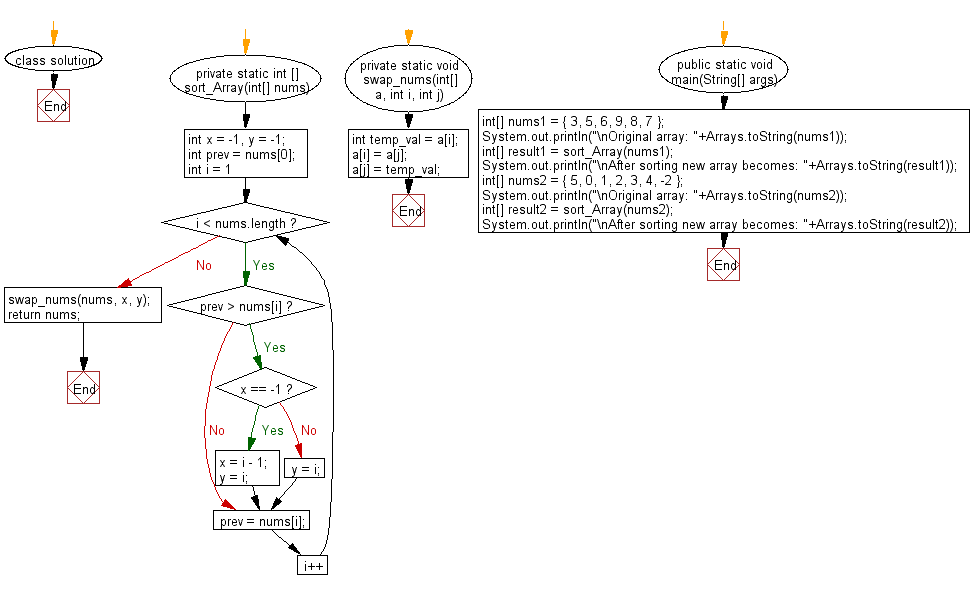﻿ Java: Sort an array of distinct integers except two numbers# Java Array Exercises: Sort a given array of distinct integers where all its numbers are sorted except two numbers

## Java Array: Exercise-73 with Solution

Write a Java program to sort a given array of distinct integers where all its numbers are sorted except two numbers.

Example:
Input :
nums1 = { 3, 5, 6, 9, 8, 7 }
nums2 = { 5, 0, 1, 2, 3, 4, -2 }
Output:
After sorting new array becomes: [3, 5, 6, 7, 8, 9]
After sorting new array becomes: [-2, 0, 1, 2, 3, 4, 5]

Sample Solution:

Java Code:

``````import java.util.Arrays;

class solution
{
private static int [] sort_Array(int[] nums)
{
int x = -1, y = -1;
int prev = nums;

for (int i = 1; i < nums.length; i++)
{
if (prev > nums[i])
{
if (x == -1) {
x = i - 1;
y = i;
}
else {
y = i;
}
}
prev = nums[i];
}

swap_nums(nums, x, y);
return nums;
}

private static void swap_nums(int[] a, int i, int j) {
int temp_val = a[i];
a[i] = a[j];
a[j] = temp_val;
}

public static void main(String[] args)
{
int[] nums1 = { 3, 5, 6, 9, 8, 7 };
System.out.println("\nOriginal array: "+Arrays.toString(nums1));
int[] result1 = sort_Array(nums1);
System.out.println("\nAfter sorting new array becomes: "+Arrays.toString(result1));
int[] nums2 = { 5, 0, 1, 2, 3, 4, -2 };
System.out.println("\nOriginal array: "+Arrays.toString(nums2));
int[] result2 = sort_Array(nums2);
System.out.println("\nAfter sorting new array becomes: "+Arrays.toString(result2));
}
}
```
```

Sample Output:

```Original array: [3, 5, 6, 9, 8, 7]

After sorting new array becomes: [3, 5, 6, 7, 8, 9]

Original array: [5, 0, 1, 2, 3, 4, -2]

After sorting new array becomes: [-2, 0, 1, 2, 3, 4, 5]
```

Flowchart:Java Code Editor:

Improve this sample solution and post your code through Disqus

What is the difficulty level of this exercise?

Test your Programming skills with w3resource's quiz.

﻿

## Java: Tips of the Day

countOccurrences

Counts the occurrences of a value in an array.

Use Arrays.stream().filter().count() to count total number of values that equals the specified value.

```public static long countOccurrences(int[] numbers, int value) {
return Arrays.stream(numbers)
.filter(number -> number == value)
.count();
}
```

Ref: https://bit.ly/3kCAgLb